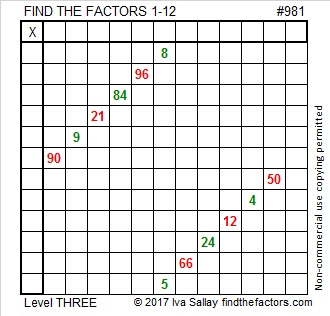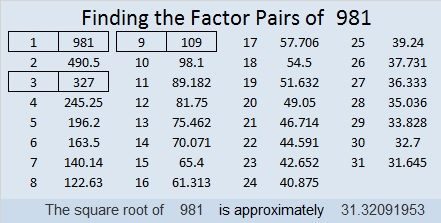# 981 Peppermint Sticks

This time of year you can buy peppermint sticks that don’t just have red stripes, but they might have green ones, too. Today’s puzzle looks like a couple of peppermint sticks. It will be a sweet experience for you to solve it, so be sure to give it a try.Print the puzzles or type the solution in this excel file: 12 factors 978-985

Here is some information about the number 981:

30² + 9² = 981 making 981 the hypotenuse of a Pythagorean triple:
540-819-981 which is 9 times (60-91-109) but can also be calculated from
2(30)(9), 30² – 9², 30² + 9²

981 is palindrome 171 in BASE 28 because 1(28²) + 7(28) + 1(1) = 981

• 981 is a composite number.
• Prime factorization: 981 = 3 × 3 × 109, which can be written 981 = 3² × 109
• The exponents in the prime factorization are 2 and 1. Adding one to each and multiplying we get (2 + 1)(1 + 1) = 3 × 2  = 6. Therefore 981 has exactly 6 factors.
• Factors of 981: 1, 3, 9, 109, 327, 981
• Factor pairs: 981 = 1 × 981, 3 × 327, or 9 × 109
• Taking the factor pair with the largest square number factor, we get √981 = (√9)(√109) = 3√109 ≈ 31.3209This site uses Akismet to reduce spam. Learn how your comment data is processed.